# Texas Go Math Grade 2 Lesson 17.3 Answer Key Centimeters and Meters

Refer to our Texas Go Math Grade 2 Answer Key Pdf to score good marks in the exams. Test yourself by practicing the problems from Texas Go Math Grade 2 Lesson 17.3 Answer Key Centimeters and Meters.

## Texas Go Math Grade 2 Lesson 17.3 Answer Key Centimeters and Meters

Essential Question
How is measuring in meters different from measuring in centimeters?
Explanation:
A centimeter is 1/100th of a meter.
It would take the length of 100 cm to equal the length of a single meter.

Explore
Draw or write to describe how you did each measurement.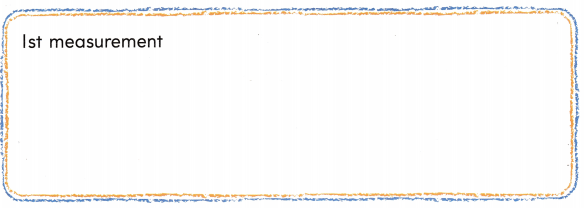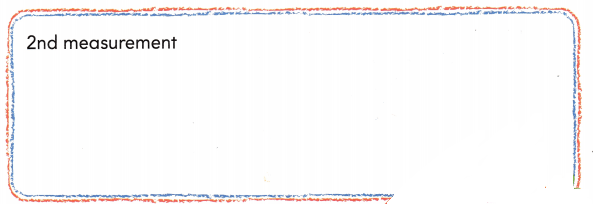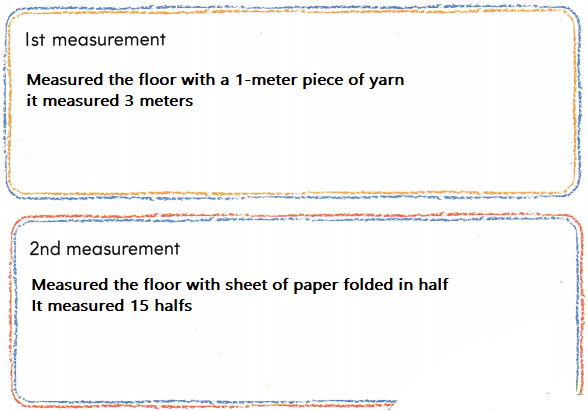Explanation:
Both the measurements are different

For The Teacher

• Have each small group use a 1-meter piece of yarn to measure a distance marked on the floor with masking tape. Then have them measure the same distance using a sheet of paper folded in half lengthwise.

Math TalK
Mathematical Processes
Describe how the lengths of the yarn and the sheet of paper are different.
Explanation:
3 x 5 = 15
1 meter is equal to half of the sheet

Model and Draw

1 meter is the same as 100 centimeters.

The real door is about 200 centimeters tall. The real door is also about 2 meters tall.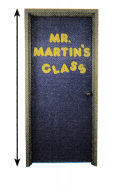Share and Show

Measure to the nearest centimeter. Then measure to the nearest meter.

Question 1.Explanation:
1 meter is the same as 100 centimeters.

Question 2.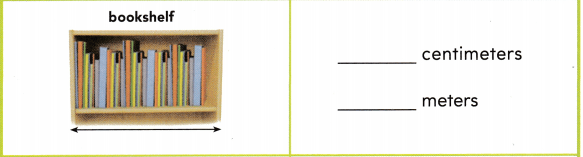Explanation:
1 meter is the same as 100 centimeters.

Question 3.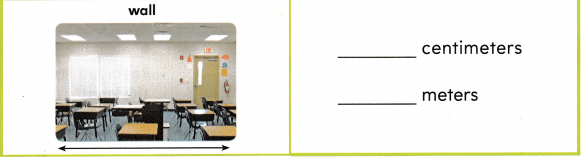Explanation:
1 meter is the same as 100 centimeters.

Problem Solving

Measure to the nearest centimeter. ‘Then measure to the nearest meter.

Question 4.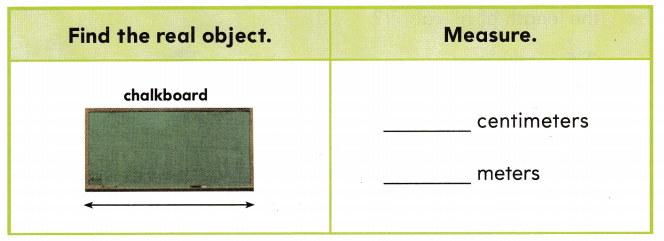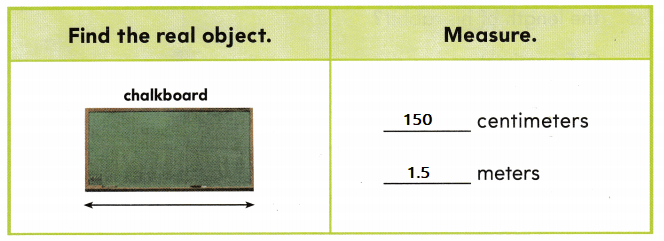Explanation:
1 meter is the same as 100 centimeters.

Question 5.
Multi-Step Mr. Ryan walked next to a barn. He wants to measure the length of the barn. Would the length be a greater number of centimeters or a greater number of meters? Explain your answer.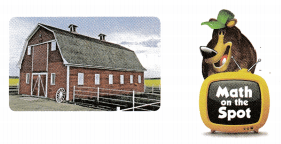Explanation:
It is very difficult to measure in centimeters

Question 6.
Write these lengths in order from shortest to longest.1 meter
200 centimeter
10 meter
Explanation:
1 meter is the same as 100 centimeters.
200 centimeter is equal 2 meters

Question 7.
Apply Adam has a pet rabbit. Which is the best choice for the length of his rabbit?
(A) 5 centimeters
(B) 4 meters
(C) 35 centimeters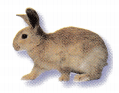Explanation:
the best choice for the length of his rabbit is 35 centimeters

Question 8.
Analyze Look at the lengths below. Which of these choices is the shortest length?
(A) 60 centimeters
(B) 6 meters
(C) 6 centimeters
Explanation:
6 centimeters is the shortest length

Question 9.
Texas Test Prep Use a centimeter ruler to measure. Which is the best choice for the length of the pencil?
(A) 20 centimeters
(B) 25 centimeters
(C) 15 centimeters
Explanation:

Take Home Activity

• Have your child describe how centimeters and meters are different.

### Texas Go Math Grade 2 Lesson 17.3 Homework and Practice Answer Key

Write the length of the paper chain to the nearest centimeter.

Question 1.___ centimeters
Explanation:
The stickers are measured on a ruler it shows the length as 14 centimeter

Problem Solving

Question 2.
Multi-Step Mr. Rojas wants to measure the length of his house. Would the length be a greater number of centimeters or a greater number of meters? Explain your answer.
Explanation:
It is very difficult to measure in centimeters

Lesson Check

Question 3.
Which is the best choice for the length of the marker?
(A) 13 meters
(B) 13 centimeters
(C) 3 centimeters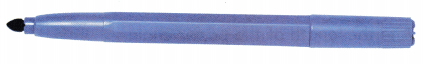Explanation:
the best choice for the length of the marker is 13 centimeters

Question 4.
Darlene sees a bull frog at a pond. What is the best choice for the length of the bull frog?
(A) 80 centimeters
(B) 8 meters
(C) 8 centimeters
Explanation:
8 centimeters is the best choice for the length of the bull frog

Question 5.
Cory has a hamster. Which is the best choice for the length of the hamster?
(A) 15 centimeters
(B) 5 meters
(C) 45 centimeters
Explanation:
15 centimeters is the best choice for the length of the hamster

Question 6.
Kate is visiting a museum. She stands next to a life-size model of a giraffe. Which is the best choice for the height of the giraffe?
(A) 6 meters
(B) 60 centimeters
(C) 6 centimeters